ChemTalk

# Half-Life## Core Concepts

In this article, you will learn what a half-life is, in 0th. 1st, and 2nd order reactions. You will also learn what the half-life formula is and how to use it to find the age of a substance.

## What is a Half-Life?

A half-life (t1/2) is the time it takes half the concentration of a sample to decay. For example, if the starting concentration of a sample is 1M, then the half-life will be the time it takes for the concentration of the sample to decay to 0.5M.

## First Order Half-Life

First-order reactions, including radioactive decay, are reactions where the rate of reaction is only dependent on the concentration of one reactant. First-order reactions have constant half-lives. We can prove this using the integrated first-order rate law: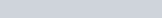[A] is the concentration of a reactant at time t, [A]₀ is the initial concentration of the reactant, and k is the rate constant. Here, we can move all the natural logs to the left side of the equation: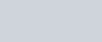Since we are trying to find the half-life of the reactant, we can set t as the half-life and [A] as half of the initial concentration: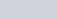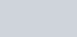Substituting those values into the equation gives us the following: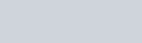Finally, dividing the k and the negative gives us an equation for the half-life of a first-order reaction: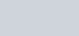Graph showing the radioactive decay of Carbon-14. Carbon-14 has a half-life of 5730 years, so the amount of Carbon-14 is cut in half after every 5730 years. Geologists often use carbon dating to find the age of rocks and organisms. Source.

## Second Order Half-Life

Second-order reactions are reactions where the rate of reaction is dependent on the concentrations of 2 reactants. The half-lives of second-order reactions depend on the reactant’s initial concentration. The integrated rate law for second-order reactions is as follows: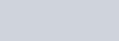Since we are trying to find the half-life of the reactant, we can set t as the half-life and [A] as half of the initial concentration:Substituting those values into the equation gives us the following: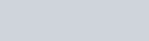We can then isolate the t to solve for the half-life of a second-order reaction: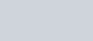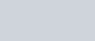## Zero Order Half-Life

In zero-order reactions, the rate of reaction does not depend on the reactant’s concentration. The half-lives of zero-order reactions, however, do depend on the initial concentration of the reactant. The integrated rate law for zero-order reactions is as follows: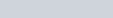Since we are trying to find the half-life of the reactant, we can set t as the half-life and [A] as half of the initial concentration:Substituting those values into the equation gives us the following: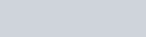We can then isolate the t to solve for the half-life of a zero-order reaction: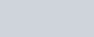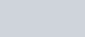## Practice Problems

1. The half-life for a first-order reaction is 2768 years. Starting with a concentration of 0.345M, what will the concentration be after 11072 years?
2. What is the half-life of a compound if 75 percent of a given sample of the compound decomposes in 60 min? Assume first-order kinetics.
3. A substance hydrolyzes in water with a rate constant of 2.0 × 10−3 min−1. Calculate the t1/2 for the hydrolysis reaction. Assume first-order kinetics.

## Solutions

1. 0.0216M. First, find out how many half-lives have passed. By dividing 11072 by 2768, we find that 4 half-lives have passed. Therefore, we can find the final concentration by dividing 0.345M by 2 four times. This leaves us with 0.0216M.
2. 30 mins. 25 percent of the sample is left, meaning 2 half-lives have passed. 60 mins divided by 2 is 30 mins, which is the half-life.
3. 350 mins. Using the equation for the half-life of a first-order reaction, we can replace k with 2.0 × 10−3 min−1.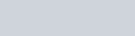We can then conclude that the half-life is 350 mins.